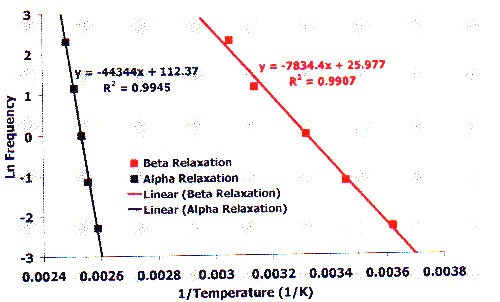Gearing Scientific Ltd., The Old Rectory, St. Lawrence, Isle of Wight, PO38 1UZ

## Summary

The use of DMA for the analysis of the α (Tg) and β relaxations of PMMA will be shown in this note. All relaxations are frequency dependant and the results of a multi frequency experiment show clear events for both the α and the β relaxations. The activation energy of both relaxations will be calculated using the Arrhenius equation.

## Introduction

Dynamic Mechanical Analysis (DMA) is one of the most appropriate methods to investigate relaxation events. The glass transition (Tg) is a key process in any material and is sometimes referred to as the a transition. Normally at a lower temperature, other relaxation events can be observed for polymeric materials. The β relaxation is normally attributed to a polymer backbone conformation reorganisation. The activation energy of these processes can be determined by performing an Arrhenius plot of the data. As frequency is essentially a rate expression with units of s-1 the natural log of frequency can be plotted against 1 over temperature. The slope of this line is equal to negative the activation energy divided by the gas constant.

#### DMA Testing

DMA works by applying an oscillating force to the material and the resultant displacement of the sample is measured. From this, the stiffness can be determined and tan 6 can be calculated. Tan 6 is the ratio of the loss component to the storage component. By measuring the phase lag in the displacement compared to the applied force it is possible to determine the damping properties of the material. Tan 6 is plotted against temperature and glass transition is normally observed as a peak since the material will absorb energy as it passes through the glass transition.

The epoxy paint samples in this experiment were run under normal temperature ramp conditions initially. To investigate the properties of the material whilst immersed in saline solution, the Triton Technology Fluid Bath was used to keep the sample immersed during the measurement.## Experimental

A bar of PMMA was clamped in the single cantilever bending clamps and cooled with LN2 to -25°C. The experiment was started collecting data at all frequencies during the same experiment.

## Results and Conclusion

The first graph on the right shows the raw tan 8 data from the experiment. Modulus data is not shown for the purposes of this application note. A clear glass transition is observed that has strong frequency dependence.

The second graph on the right shows an expanded and enlarged section from -40°C to 80°C. As well as being expanded, a baseline subtraction was also applied. This is because the glass transition overlaps the ß relaxation to a small degree. When the whole glass transition is subtracted from the data, the second graph on the right is produced. It is clear that a β relaxation is visible. Again, this is frequency dependant as expected for a relaxation event.

#### PMMA Testing

The Arrhenius equation can be expressed as: Arrhenius Equation where F is frequency, A is the preexponential factor, Ea is the activation energy, R is the gas constant and T is temperature. A plot of Ln frequency against 1/temperature is known as an Arrhenius plot.

#### PMMA Testing

The final graph shows the Arrhenius plot with a line of best fit and the equation of that line.

The higher temperature glass transition data is shown in black and the lower temperature β relaxation is shown in red. The slope of each line is equal to negative the activation energy divided by the gas constant. The activation energy for the β relaxation of PMMA is calculated to be 65kJmol-1 and for the glass transition is 368 kJmol-1. Both lines show excellent fit parameters.